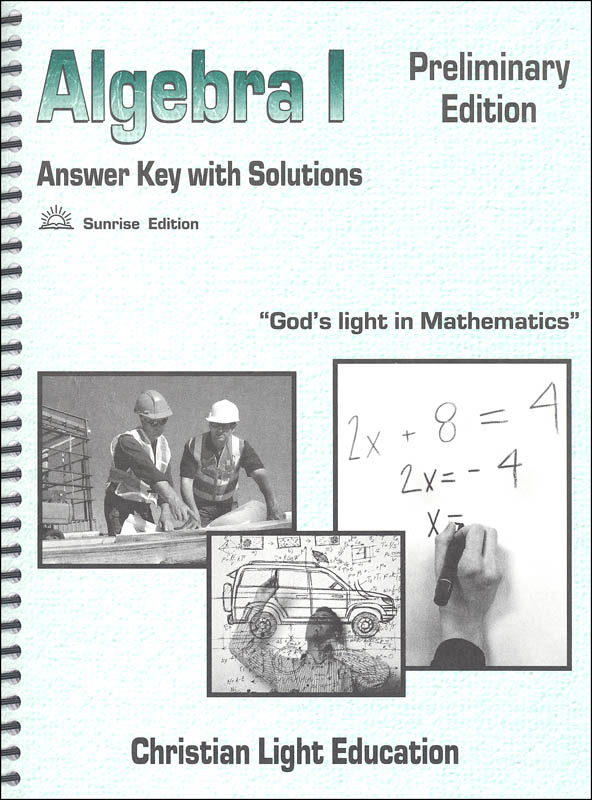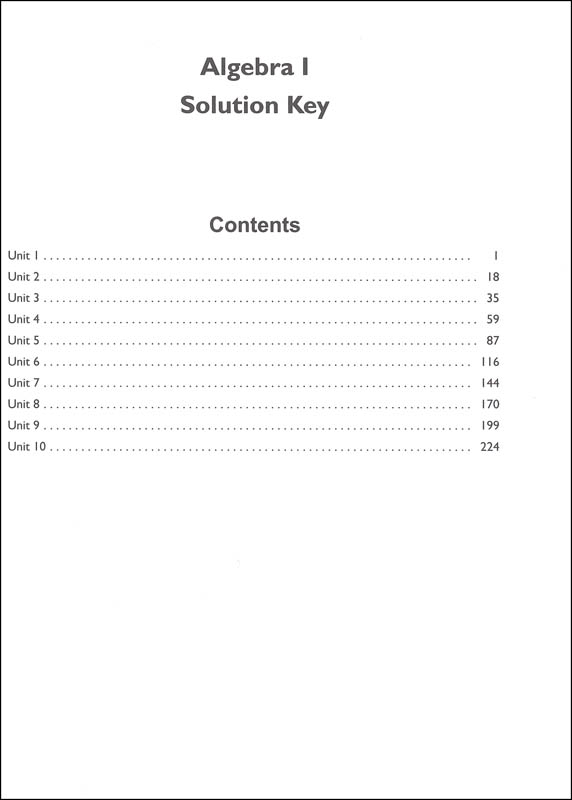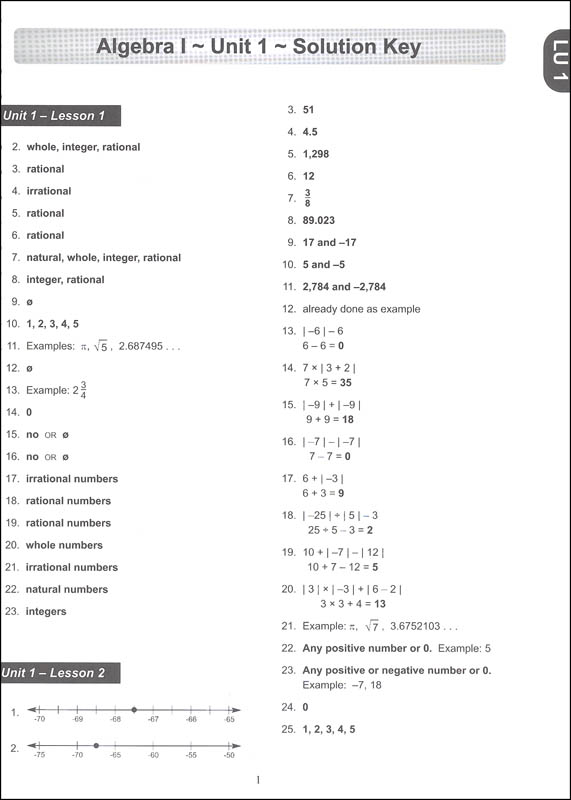•••# Algebra I Teacher's Guide/Solution Key with answers Sunrise Edition, Preliminary

# 072147

Our Price: \$10.00
In Stock.
Qty:
Qty:

 Item #: 072147 9

#### Publisher Description:

This solution key contains solutions and answers for the student work for the Sunrise Preliminary Algebra 1 course.

Category Description for Algebra I (CLE Math):

Offering an incremental, spiral approach, CLE Algebra offers a consistent systematic review to help students thoroughly learn the concepts. Topically, students learn absolute value, variables, ratios and percents, exponents, roots and radicals, polynomials, radicals, binomials, graphing, linear algebra, quadratic equations, probability and much more. You may choose one of two options for teaching this course: a full-color, hardcover Textbook or the reusable soft cover Units. The same scope of concepts is covered. The Units use the same methodology as the elementary Sunrise Math. Textbook lessons have been edited for clarity, and user friendliness. Text lessons use the same Sunrise review and an incremental approach to teaching. Each lesson begins with a new concept explanation and examples. Students then complete “Today’s lesson” problems to practice the new concept. Review problems from previous lessons follow. Small reference numbers are included to identify the lesson where the concept was previously taught. Lessons conclude with a few additional practice problems related to the new concept taught in the lesson. Lessons 5,10, and 16 of every unit are quiz and test lessons, and include an optional enrichment activity. Compared to the Unit lessons, the Textbook lessons are sequenced differently and are not interchangeable. Either option requires the purchase of separate tests and quizzes, answer keys and/or solutions. Necessary components for the Textbook Approach include the Textbook, the Solutions Key 1-5 and 6-10, and the Tests & Quizzes. The Solutions Keys include solutions to the textbook and the tests and quizzes. Components for the Unit approach are the set of 10 reusable units (students write on a separate piece of paper), a quiz and test packet, a quiz and test answer key, and the Solutions Guide for the Units. Please note the publisher refers to the units as Preliminary Units. The publisher plans on the preliminary edition being available for several years until they refine the materials into a full color textbook. ~ Deanne

Category Description for Christian Light Mathematics:

To help ensure students learn their math facts, daily speed drills, oral exercises, and flash-cards are tools used for students in grades 1-4. Basic algebraic and geometric concepts are introduced early, along with money, problem solving, mental math, and number patterns to help students become proficient in these skills. A few supplemental materials are needed for the lower grades Grade 1 uses addition and subtraction flash cards (#72144); My Counting Book (#72357); Counting Chart (#72173); and optional My Calendar Book (#21786); and student clock (#15339 or #60101); Grade 2 uses addition and subtraction flash cards (#72144); Counting Chart (#72173); and optional multiplication/division flash cards (#72356); My Calendar Book (#21786); student clock (#15339 or #60101); and math reference chart (#68518); Grade 3 uses optional flash cards as in 1st and 2nd grades, and the math reference chart. Grades 4, 5, and soon to be 6 (later in 2018) offer the option of using the Light Unit approach or text book approach same content but a choice of consumable or non-consumable.

If you choose the Light Units path, parents need only to purchase the Light Units and Teacher Guides or answer keys. If choosing the textbook path, then you will need the textbook (non-consumable), student pack (consumable), Teacher Guide and/or Full Solutions Answer Key. Textbooks are colorful with illustrations and pictures, and provide opportunity for students to do their work on a separate sheet of paper. The Student Pack includes quizzes, tests, and daily speed/mastery drills (math facts review) and measurement equivalents. Full Solutions Answer Key shows each problem of the textbook, quizzes, and tests with step-by-step solutions. Teacher Guides for the textbook are also in full color and include student pages with answers and a cd of printables posters, charts, and practice sheets.

The addition/subtraction flash cards accompanying this curriculum for grades 1-4 are numbered and tabbed to correspond to the teacher guides at those grade levels. Cards measure 3.5" x 7.5", addition/subtraction go from 0 to 18. Multiplication/division flash cards measure 3.5" x 5" and cover math facts from 0-12. The multiplication fact is on the front of the card with the opposite division fact on the reverse. These aren't numbered and tabbed like the addition/subtraction cards. Math Reference Charts are available for grades 2-6, 5-8, and 7/8. These are 8.5" x 11" laminated sheets for easy reference at a glance for those items that students need to remember but need a little help measurement tables, formulas, etc.

Grade 8 is considered pre-algebra and prepares students for any algebra 1 course. It introduces students to concepts needed at the higher levels of math such as Pythagorean theorem, irrational square roots, and basic trigonometry.

The sequence for high school is Algebra 1, Geometry, Algebra II, and Functions and Trigonometry. To use Algebra I you will need the 10 Light Units (students write on their own paper), solution key (solutions to the Light Units exercises), tests & quizzes, and the test & quiz answer key. These are all sold separately for the Algebra I level. Geometry, Algebra II, and Functions and Trigonometry, each use 10 Light Units and 5 answer keys. Finishing through Functions and Trigonometry will prepare students for college level math.

Consumer Math is an optional math course offered in this series. Equivalent to one high school credit, this course applies math to everyday situations. There are 10 Light Units and 5 answer keys needed to complete the course and they cover basic and everyday math fractions, decimals, percent, family finances, construction & building trades, service occupations, transportation, business services, and occupational diagrams. ~ Donna

Category Description for COMPREHENSIVE PROGRAMS - ALL GRADES:

Primary Subject
Mathematics
9
Format
Paperback
Brand Name
Christian Light
Weight
1.45 (lbs.)
Dimensions
10.62" x 8.5" x 0.75"